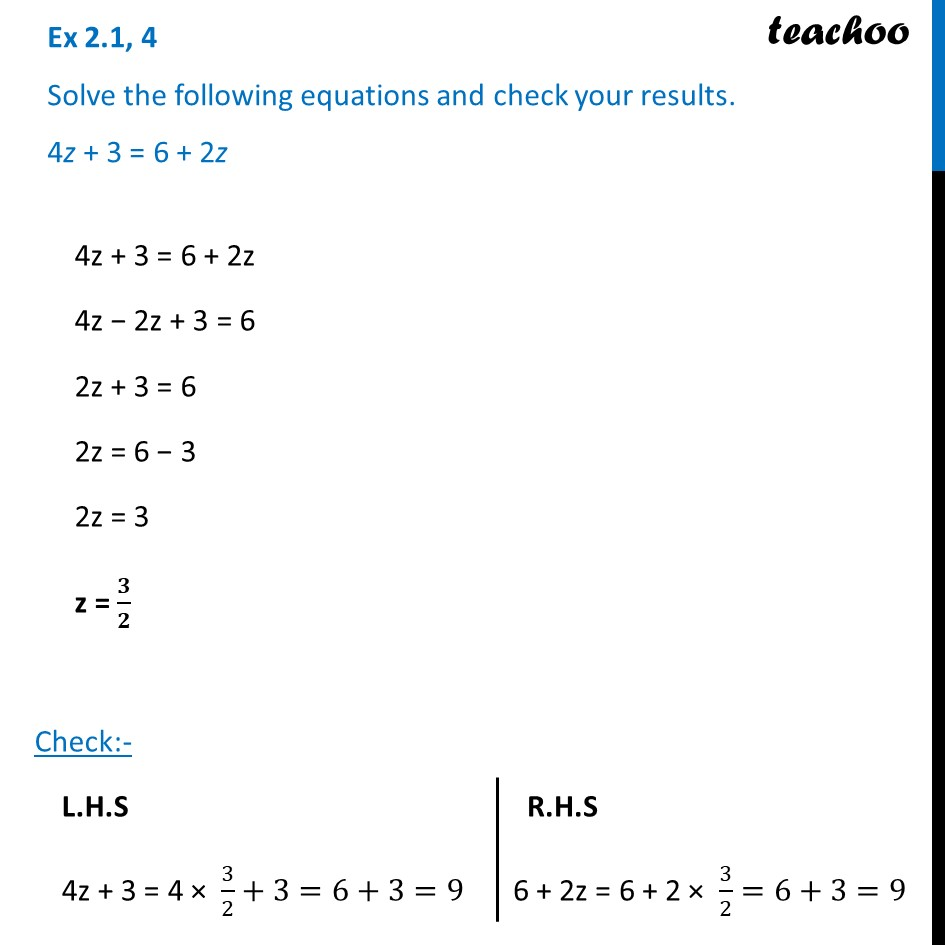Ex 2.1

Chapter 2 Class 8 Linear Equations in One Variable
Serial order wiseLearn in your speed, with individual attention - Teachoo Maths 1-on-1 Class

### Transcript

Ex 2.1, 4 Solve the following equations and check your results. 4z + 3 = 6 + 2z4z + 3 = 6 + 2z 4z − 2z + 3 = 6 2z + 3 = 6 2z = 6 − 3 2z = 3 z = 𝟑/𝟐 Check:- L.H.S 4z + 3 = 4 × 3/2+3=6+3=9 R.H.S 6 + 2z = 6 + 2 × 3/2=6+3=9 ∴ LHS = RHS Hence Verified.